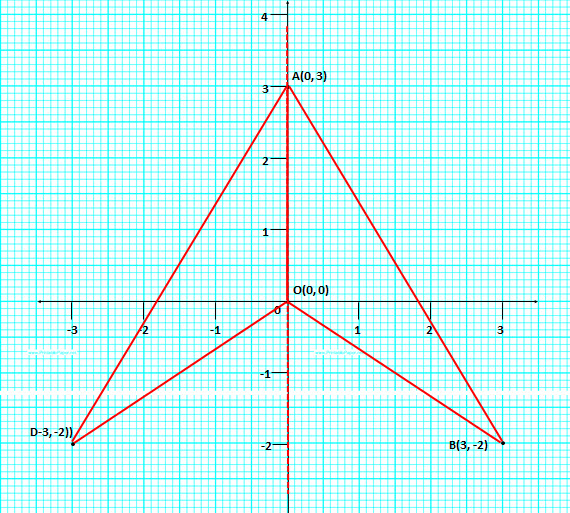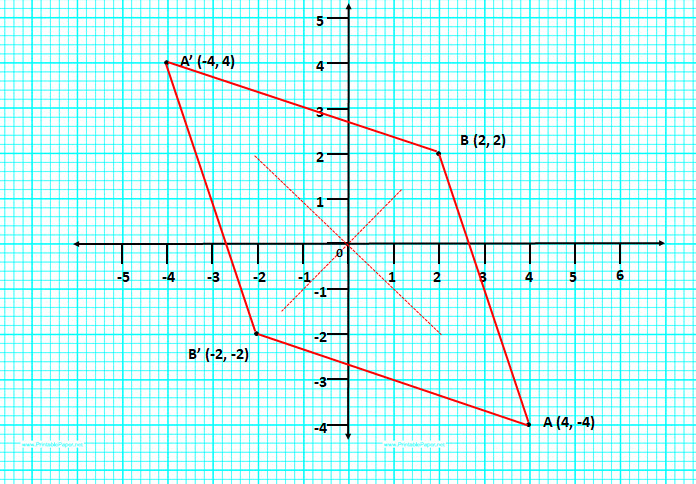Question 1: Use graph paper for this question. Plot the points$A (8, 2) \ and \ B (6, 4)$. These points are the vertices of a figure which is symmetrical about$x = 6 \ and \ y = 2$. Complete the figure on the graph. Write down the geometrical name of the figure.   [2000, 2006]Name of the geometrical figure is a square as all the sides are equal and parallel.$\\$

Question 2: Use graph paper for this question. Use 2 cm = 1 unit on both the axes. Plot the points$A (-2, 4), B (2, 1) \ and \ C (-6, 1)$.

(i) Draw the line of symmetry of$\triangle ABC$.

(ii) Mark the point$D$ if the line in (i) and the line$BC$ are both lines of symmetry of the quadrilateral$ABCD$. Write down the co-ordinates of point$D$.

(iii) What kind of quadrilateral is figure$ABCD$?

(iv) Write down the equation of$BC$ and the line of symmetry named in (i.)(i) The dotted line is the line of symmetry. The equation of that line is$y = 1 \ and \ x = -2$

(ii) Coordinates of point$D = (-2, -2)$

(iii) It is a Rhombus.

(iv) The equation of that line is$y = 1 \ and \ x = -2$$\\$

Question 3: Use graph paper for this question$A (0, 3), B (3, -2) \ and \ O (0, 0)$ are the vertices of$\triangle ABO$.

(i) Plot$D$ the reflection of$B$ in the$y-axis$, and write its co-ordinates.

(ii) Give the geometrical name of the figure$ABOD$.

(iii) Write the equation of the line of symmetry of the line$ABOD$.(i)  Plotted above

(ii) The coordinates of$D (-3, -2)$

(iv)$x = 0$ is the equation of line of symmetry$\\$

Question 4: Using graph paper and taking 1 cm = 1 unit along both$x \ and \ y axis$.

(i) Plot the points$A (-4, 4) \ and \ B (2, 2)$.

(ii) Reflect$A \ and \ B$ in the origin to get the images$A' \ and \ B'$ respectively.

(iii) Write down the co-ordinates of$A' \ and \ B'$.

(iv) Give the geometrical name for the figure$ABA'B'$.

(v) Draw and name its lines of symmetry.(i) Plotted above.

(ii) Marked$A' \ and \ B'$

(iii)$A'(-4, 4) \ and \ B'(-2, -2)$

(iv) Lines of symmetry are$y = x \ and \ y = -x$$\\$

Question 5: Use graph paper to answer the following questions. (take 2 cm = 1 unit on both the axes)

(i) Plot the points$A (-4, 2)$ and$B (2, 4)$.

(ii)$A'$ is the images of$A$ reflected in the$y-axis$. Plot it on the graph paper and write the co-ordinates of$A'$.

(iii)$B'$ is the image of$B$ when reflected in the line$AA'$. Write the co-ordinates of$B'$.

(iv) Write the geometrical name of the figure$ABA'B'$

(v) Name a line of symmetry of the figure formed.(i) Plotted above

(ii)$A'(4, 2)$

(iii)$B'(2,0)$

(iv) The figure is a kite

(v)$y=2$ is a line of symmetry$\\$